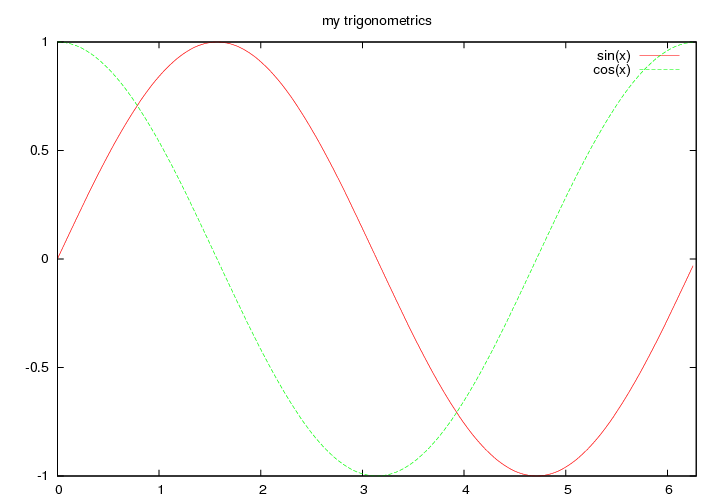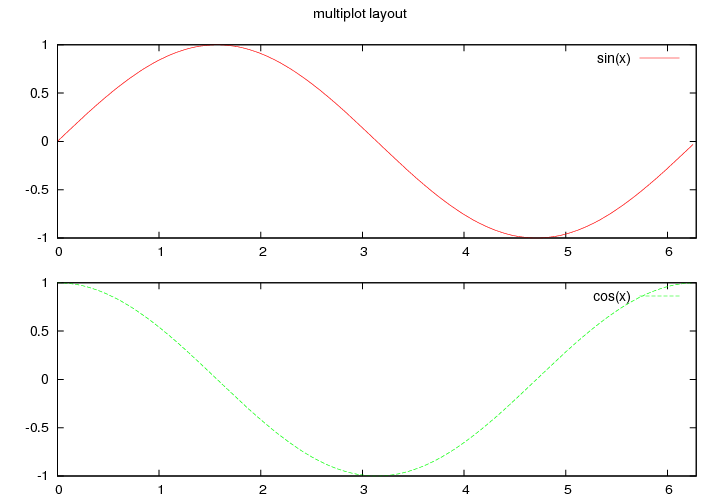Version: 4.1.3.1

## gnuplot.plt

### 1Overview

This library provides a wrapper interface to gnuplot. Using the library, mzscheme programs can spawn inferior gnuplot processes and plot data to windows or hardcopy with a programmatic interface.

The library assumes gnuplot version 4.x (tested with 4.2.3).

(C) Copyright 2008 Dimitris Vyzovitis <vyzo at media.mit.edu>

gnuplot.plt is free software: you can redistribute it and/or modify it under the terms of the GNU General Public License as published by the Free Software Foundation, either version 3 of the License, or (at your option) any later version.

gnuplot.plt is distributed in the hope that it will be useful, but WITHOUT ANY WARRANTY; without even the implied warranty of MERCHANTABILITY or FITNESS FOR A PARTICULAR PURPOSE. See the GNU General Public License for more details.

You should have received a copy of the GNU General Public License along with gnuplot.plt. If not, see <http://www.gnu.org/licenses/>.

### 2API

#### 2.1Gnuplot processes

 (gnuplot-spawn [read?]) → gnuplot? read? : bool? = #f
 (gnuplot-kill gplot) → any gplot : gnuplot?
 (gnuplot? o) → bool? o : any
 (gnuplot-input-port o) → input-port? o : gnuplot?
 (gnuplot-writeln o fmt arg ...) → any o : gnuplot? fmt : string? arg : any

gnuplot-spawn spawns a gnuplot processes, using (gnuplot-program).

When read? is #t then output from gnuplot can be collected through the port accessible with gnuplot-input-port. gnuplot-writeln can be used to write directly to the process’s input port.

The processes is garbage collected when unreachable, but can be explicitly killed with gnuplot-kill

 (gnuplot-program) → path-string?
 (gnuplot-set-program! o) → any o : path-string?

The gnuplot executable.

Defaults to "gnuplot" under unix/macosx and "pgnuplot.exe" under windows.

#### 2.2Data sources

 (gnuplot-data data [ #:file fname #:comments comments #:tmpdir tmpdir #:binary bin?]) → gnuplot-data?
data : list?
fname : path-string? = #f
comments : list? = #f
tmpdir : path-string? = #f
bin? : bool? = #f

Creates a data source using data, which must be a list of lists of numbers, written to a temporary file when fname is #f.

When bin? is #f files are written in ascii, optionally prepending comments from comments which when supplied must be a list of strings. When bin? is #t data is written in binary form, following gnuplot’s binary matrix file format.

Temporary files are automatically deleted when the data sources become unreachable.

 (gnuplot-data/file fname [bin?]) → gnuplot-data? fname : path-string? bin? : bool? = #f

Create a data source using an existing file.

 (gnuplot-data? o) → bool? o : any

#t when o is a gnuplot data source.

#### 2.3Plotting

Plotting is controlled using a simple translation scheme that maps items to gnuplot’s idiosyncratic syntax, with the following grammar:

 source-item = (item   ...) sequence-item = (seq  ...) | (seq:  ...) | (seq*  ...) range-item = () | (/#f /#f) | ( /#f /#f) | param-item = (=  ) path-item = (path ) str-item = (str ) empty-item = #f any-item =

• source-item – is a plot directive.

• sequence-item – is mapped to a sequence of items, joined with ",", ":", or " " respectively.

• range-item – is a plot range.

• param-item – is a parametric expression.

• path-item – is a single quoted string, while a str-item is a double-quoted string.

• empty-item – is translated to an empty string

• Any other object is translated to its printed representation using format.

 (gnuplot-item data [options]) → item? data : gnuplot-data? options : plot-item? = null

Create a plot item, using data as the data source, prepended to options.

 (gnuplot-plot gplot [#:range range] item ...) → any gplot : gnuplot? range : list? = #f item : item?
 (gnuplot-splot gplot [#:range range] item ...) → any gplot : gnuplot? range : list? = #f item : item?
 (gnuplot-replot gplot item ...) → any gplot : gnuplot? item : item?

Basic plotting functions.

range, when present, must be a list of range items.

 (gnuplot-multiplot gplot [ #:layout layout] plot ...) → any
gplot : gnuplot?
layout : list? = null
plot : procedure?

Performs a multiplot, with layout a list of items, by calling the plot procedures in order with gplot as argument.

 (gnuplot-hardcopy gplot fname [ plot #:term term]) → any
gplot : gnuplot?
fname : path-string?
plot : procedure? = gnuplot-replot
term : item? = '(postscript color)

Plots with output to file.

 (gnuplot-set gplot opt) → any gplot : gnuplot? opt : item?
 (gnuplot-set* gplot opt) → any gplot : gnuplot? opt : list?
 (gnuplot-unset gplot opt) → any gplot : gnuplot? opt : symbol?
 (gnuplot-reset gplot) → any gplot : gnuplot?

Modify plot options.

### 3Example

The images were created with gnuplot-hardcopy and converted to png using imagemagick’s convert utility

Here we use a simple iterative example to illustrate how to use the library, assuming familiarity with gnuplot.

First, let’s create a gnuplot process and a simple data source:

 (define gplot (gnuplot-spawn)) (define sin+cos (build-list 200 (lambda (x) (let ((x (/ (* x pi) 100))) (list x (sin x) (cos x)))))) (define mydata (gnuplot-data sin+cos))

Here, mydata is a data source that contains 200 samples from the sin and cos functions.

We can split the two functions, creating two plot items:

 (define mysin (gnuplot-item mydata '(using (seq: 1 2) title (str "sin(x)") with line 1))) (define mycos (gnuplot-item mydata '(using (seq: 1 3) title (str "cos(x)") with line 2)))

Now, to plot the two functions together:

 (gnuplot-set gplot '(title (str "my trigonometrics"))) (gnuplot-plot gplot #:range `((#f ,(* 2 pi)) ()) mysin mycos)We can take a hardcopy of the plot using gnuplot-hardcopy:

(gnuplot-hardcopy gplot "/tmp/trig.eps")

We can create a multiplot layout using gnuplot-multiplot:

 (define (plot-sin gplot) (gnuplot-plot gplot #:range `((#f ,(* 2 pi)) ()) mysin)) (define (plot-cos gplot) (gnuplot-plot gplot #:range `((#f ,(* 2 pi)) ()) mycos)) (gnuplot-reset gplot) ; reset plotting (gnuplot-multiplot gplot #:layout '(layout (seq 2 1) title (str "multiplot layout")) plot-sin plot-cos)Finally, to take a hard copy of the multiplot layout:

 (gnuplot-hardcopy gplot "/tmp/multi.eps" (lambda (gplot) (gnuplot-multiplot gplot #:layout '(layout (seq 2 1) title (str "multiplot layout")) plot-sin plot-cos)))